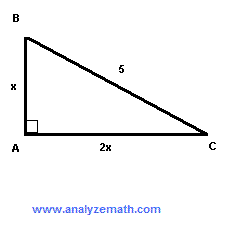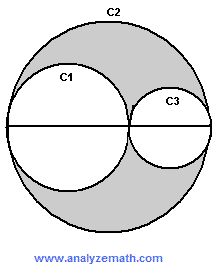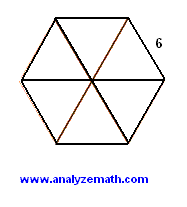Free Compass Math Test Practice Questions with Answers - Sample 1

Math questions, with answers, similar to the questions in the compass math test are presented. The questions are designed to reflect the major topics covered in the compass test: Numerical skills/pre-Algebra, algebra, college algebra, geometry and trigonometry. The answers are at the bottom of the page and the solutions with full explanations to these questions are also included.

A - Numerical Skills/Pre-Algebra

1.A) 21
B) 23
C) 44
D) 68
E) 74

2. In scientific notation, 3.0 x 10-5 + 0.0000022 =

A) 3.5 x 10-5
B) 3.22 x 10-5
C) 5.2 x 10-6
D) 3.5 x 10-6
E) 5.2 x 10-11

3. If.

is calculated and the fraction obtained reduced to simplest form, then what is its numerator?
A) 12
B) 8
C) 7
D) 6
E) 3

4..

A) 59/80
B) 17/20
C) 6/7
D) 16/15
E) 17/15

5. A shop owner increased the selling price of a shirt from $20 to$26. By what percentage was the price increased?
A) 6
B) 26
C) 30
D) 46
E) 60

6. 3/4+0.85+20% =

A) 27.85
B) 1.8
C) 23.85
D) 2.85
E) 21.6

7. Tom worked for 6 hours and was paid $5.50 per hour. He then went shopping and bought two magazines at$9.50 each and a pen at $8.25. How much money does Tom have left? A)$60.25
B) $15.25 C)$33
D) $27.25 E)$5.75

8. Bill bought 1(3/4) pounds of Cheddar cheese, 3.75 pounds of blue cheese and 4(1/2) pounds of goat cheese. How many pounds of cheese did Bill buy?
A) 8
B) 7.75
C) 9.25
D) 10
E) 7

9. In a certain school, 40% of all students are 8 years old or younger. The number of the remaining students is 120. How many students are 8 years old or younger?
A) 80
B) 160
C) 480
D) 320
E) 740

10. 1/5 of students in a school have no brothers or sisters. Of the remaining students, 40% have 1 brother or sister. What percent of all students have more than 1 brother or sister?
A) 60
B) 80
C) 48
D) 20
E) 45

11. A car hire company offers two plans A and B. In plan A, a customer pays a flat fee of $20 for the day plus 5 cents for each kilometer. In plan B, a customer pays a flat fee of$15 for the day plus 7 cents for each kilometer. For how many kilometers would a customer pay the same amount for the two plans?
A) 1000
B) 800
C) 242
D) 400
E) 250

12. Joe worked part time for x hours and earned y dollars. How much does he earn if he works z hours?
A) yz/x
B) z/(xy)
C) xyz
D) zx/y
E) xy/z

13. In a science test, a class of 30 students had an average of 80. The 20 girls in the class had an average of 85. What is the average of the 10 boys in the class?
A) 60
B) 70
C) 80
D) 85
E) 90

14. Find y if y / 5 = 10 / 25.
A) 80
B) 25
C) 2
D) 8
E) 1

15. √(32 + 42) =
A) 7
B) 25
C) 50
D) 12
E) 5

B - Algebra

16. If a = -2 and b = -2, what is the value of (a3 - 1) / (b - 1)
A) 3
B) -9
C) -3
D) 9
E) 6

17. If -2(x + 9) = 20, then - 4x =
A) -76
B) -19
C) 0
D) 76
E) 90

18. x is a variable such that if 20% of it is added to its fifth the result is equal to 12 subtracted from seven tenths of x. Find x.
A) 0.2
B) 1/5
C) 7/10
D) 20
E) 40

19. The area A of a trapezoid is given by the formula A = 0.5(b + B)h, where b and B are the sizes of the bases and h the size of the height of the trapezoid. Express B in terms of A, b and h.
A) 0.5Ah - b
B) 2A/h - b
C) (2A - b)/h
D) 2A/h + b
E) (2A + b)/h

20. Tom, Linda and Alex have \$120 dollars. Alex has the third of what Tom has and Linda has twice as much as Alex. How much money, in dollars, does Linda have?
A) 10
B) 20
C) 40
D) 60
E) 80

21. Which of the following is equivalen to 6x2 - 11x - 2?
A) (6x - 1)(x + 2)
B) (3x - 1)(2x + 2)
C) (3x + 1)(2x - 2)
D) (6x + 1)(x - 2)
E) (x + 1)(6x - 2)

22. Which of the following is a factor of x2 - 7x - 8?
A) x + 1
B) x + 8
C) x + 7
D) x - 1
E) x - 7

23. (2xy2 - 3x2y) - (2x2y2 - 4x2y) =
A) 2x2y2
B) -2x2y2 -7x2y - 2x2y2
C) -2x2y2 + x2y - 2x2y2
D) 2x2y2 + x2y - 2x2y2
E) -2x2y2 + x2y + 2xy2

24. During the same journey, Stuart drove x miles for 2 hours, and 200 miles for 3 hours. Find x if the average speed for the entire journey is 70 miles per hour.
A) 166
B) 167
C) 150
D) 140
E) 120

25. Given the equations of the lines
(I) 2y + 3x = 3
(II) -3y - 2x = 5
(III) -6y + 4x = 9,
(IV) 2y + 6x = 9
which two lines are perpendicular?

A) (I) and (II)
B) (II) and (III)
C) (III) and (IV)
D) (I) and (III)
E) (IV) and (II)

26. If f(x) = (x + 1)2, then f(t + 2) =
A) t2 + 2t + 4
B) t2 + 4
C) t2 + 6t + 9
D) t2 + 9
E) t2 + 2t + 1

27. For x > 0 and y > 0,
(√x + √y) (√x - √y) - (√x - √y)2 =

A) -2√x√y - 2y
B) x - y
C) 0
D) -2√x√y + 2y
E) 2√x√y - 2y

28. What is the slope of the line whose equation is given by
x/2 - y/4 = 7

A) 2
B) 1/2
C) -1/4
D) -1/2
E) -2

29. For x > 3,
(x / (x - 3) + 1 / 2)(2/(x - 1)) =

A) (x + 1) / ((x - 3)(x - 1))
B) 3 / (x - 3)
C) (x + 3) / (2(x - 1))
D) 2x / ((x - 3)(x - 1))
E) 1 / (x - 3)

30. In a standard rectangular system of axes, point A has the coordinates (2 , 1). What must be the coordinates of point B if M(3 , 2) is the midpoint of the segment AB?

A) (1 , 1)
B) (4 , 3)
C) (3 , 4)
D) (3 , 2)
E) (2 , 3)

C - College Algebra

31. What is the value of X in the following geometric sequence
X , 64 , ___ , ___ , 8

A) 1/2
B) 32
C) 256
D) 128
E) 512

32. For what value of K will the system of equations given below have no solution?

2x + 5y = 9
-3x - K y = 4

A) 5
B) -5/2
C) 15/2
D) 5/2
E) 17/2

33. If f(x) and g(x) are two functions defined by

f(x) = √(x + 1)
g(x) = | x - 1 |,

then what is the value of f(g(9))?
A) 8√10
B) | √10 - 1 |
C) 3√10
D) -3
E) 3

34. The range of

f(x) = x2 -2x + 1
is given by the interval

A) [0 , +infinity)
B) (-infinity , 1]
C) [1 , +infinity)
D) (0 , + infinity)
E) (1 , + infinity)

35. If f(x) is a linear function such that f(-1) = 11 and f(2) = 5,
then f(0) =

A) 5
B) 6
C) 7
D) 8
E) 9

36. Which of these functions is(are) always increasing?
(I) f(x) = -x2 + 2x + 1
(II) f(x) = 2x - 1000
(III) f(x) = |x - 4| + 255
(IV) f(x) = -e-x,

A) (I) and (II) only
B) (II) only
C) (II) and (IV) only
D) (I) and (III) only
E) (III) and (IV) only

37. i is the imaginary unit such that i = √-1. What is the value if i99?
A) i
B) -i
C) 1
D) -1
E) 99

38. If.

Find a, b and c.

A) 2 , -1 , 3
B) 1 , 2 , 3
C) 3 , -2 , 1
D) 1 , -2 , 3
E) 1 , 2 , -3

39. In an arithmetic series, the 3rd term is equal to 114 and the last term is equal to -27. The sum of all terms in the series is equal to 2325. What are the last three terms in the series?

A) -13 , -20 , -27
B) -7 , -17 , -27
C) -25 , -26 , -27
D) 27 , 0 , -27
E) -21 , -24 , -27

D - Geometry

40. In the figure below, line T1 is parallel to line T2 and line L1 is parallel to line L2. The pair of lines T1 , T2 are not perpendicular to L1,L2. Which of the set of angles below has all 4 angles equal in measurement?.

A) {a,b,c,d}
B) {e,f,g,h}
C) {e,f,m,h}
D) {j,k,f,g}
E) {a,b,f,g}

41. A cube with edge length x and a right cylinder with circular base have the same volume. Find x ,in cm, if the radius of the cylinder is 5 cm and its height is 5 cm.

A) 5(Pi)1/3
B) 51/3
C) 5Pi
D) 125Pi
E) 125

42. Find, in square cm, the area of an equilateral triangle of side 20 cm.

A) 400
B) 200
C) 200√3
D) 100√3
E) 50√3

43. The two circles shown below have diameters AB and BD and are tangent at B. ABC and BDE are isosceles triangles inscribed inside the circles. What is the measurement, in degrees, of angle CBE?.

A) 135
B) 90
C) 85
D) 75
E) 45

44. In the figure below, EB and FC are both perpendicular to AC and x is the length of AB. If the length of AC is 11, what is x?.

A) 9/4
B) 4/9
C) 44/9
D) 4
E) 99/4

45. ABC is a right triangle such that the length of BC is 5 units. The legs have length x and 2x. Find x..

A) 1
B) 2
C) 5√5
D) √5
E) 2√5

46. AB is tangent to the circle with center O and radius 10 cm. The length of OA is 15 cm and the length of OB is 20 cm. Find the area, in square cm, of triangle ABO..

A) 300
B) 150
C) 100√5 + 50√3
D) 100(√5 + √3)
E) 25√5 + 50√3

47. The area of the trapezoid shown below is 128 square cm. Find x in cm..

A) 8
B) 9
C) 10
D) 11
E) 12

48. C1, C2 and C3 are three tangent circles with their centers on the same line. If R is the radius of the large circle and the diameter of circle C1 is twice the diameter of circle C3, then what is the area of the shaded region?.

A) (4/9)R2Pi
B) (5/9)R2Pi
C) (1/3)R2Pi
D) (1/2)R2Pi
E) (7/9)R2Pi

E - Trigonometry

49. If x is a positive angle smaller than 90� and cos(x) = 1/3, then sin(x) =

A) 2/3
B) 2√2 / 3
C) √2
D) 8/9
E) 4/9

A) Pi/4
B) Pi/3
C) 3Pi/4
D) Pi/6
E) 2Pi/3

51. The measurement of an angle of a right triangle is 40� and the leg opposite to this angle is 5 cm long. What is the length, in cm, of the other leg?

A) 5/tan(50)
B) tan(50)/5
C) tan(40)/4
D) 5/tan(40)
E) 4/tan(40)

52. Which of these graphs is the graph of y = cos(2x)?.

53. What is the smallest positive value of x for which y = cos(3x) has a minimum value?

A) Pi
B) Pi/2
C) Pi/4
D) Pi/6
E) Pi/3

54. If you put 6 equilateral triangles together as shown below, you can make a regular hexagon. What is the area, in square units, of a regular hexagon of side lenght 6 units?.

A) 54√3
B) 9√3
C) 36
D) 6√3
E) √3